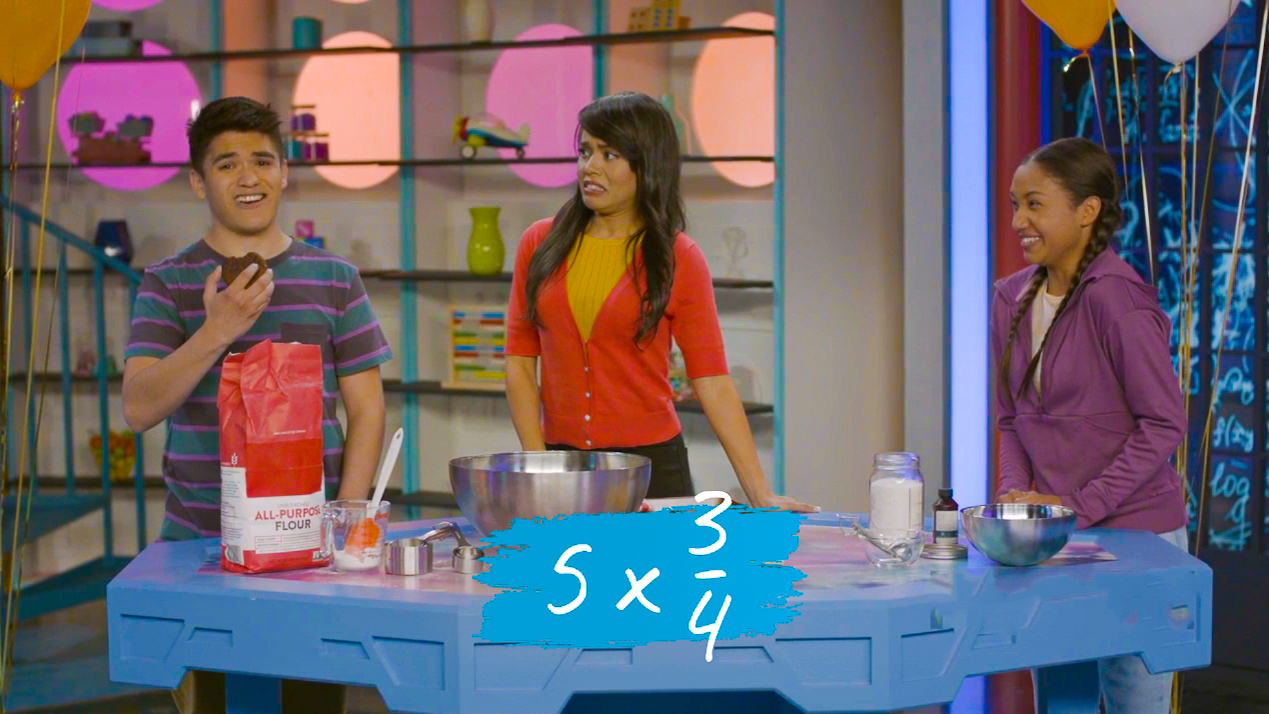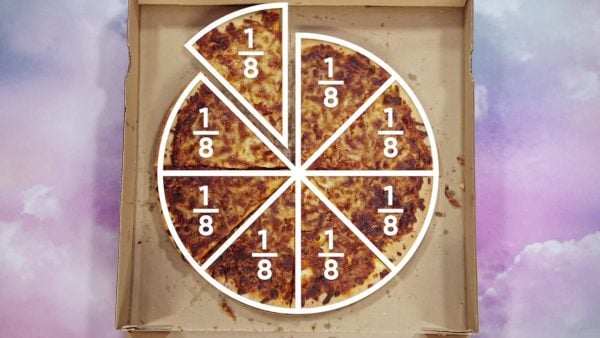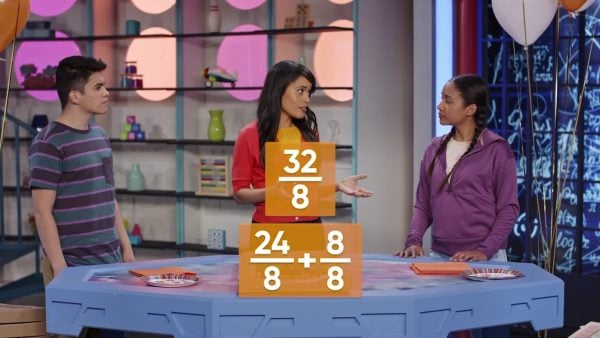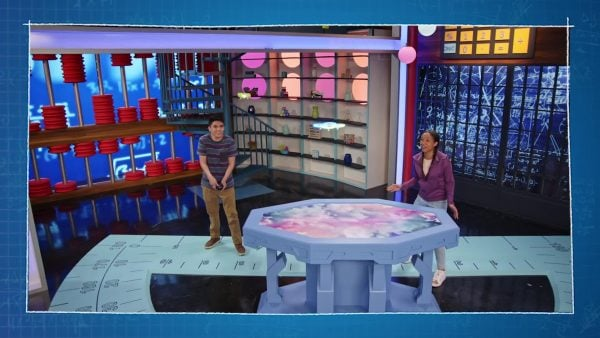Multiplying Fractions by Whole Numbers Reading Material | Generation Genius
1%
It was processed successfully!

# Multiplying Fractions by Whole NumbersWHAT IS MULTIPLYING FRACTIONS BY WHOLE NUMBERS?

Students connect repeated addition of fractions to multiplying fractions by whole numbers. After exploring examples in many different contexts where a fraction is multiplied by a whole number, they develop the following rule: Multiply the whole number by the fraction’s numerator and keep the fraction’s denominator.

To better understand multiplying fractions by whole numbers…

WHAT IS MULTIPLYING FRACTIONS BY WHOLE NUMBERS?. Students connect repeated addition of fractions to multiplying fractions by whole numbers. After exploring examples in many different contexts where a fraction is multiplied by a whole number, they develop the following rule: Multiply the whole number by the fraction’s numerator and keep the fraction’s denominator. To better understand multiplying fractions by whole numbers…

## LET’S BREAK IT DOWN!

### Planning a Pizza Party for 8 PeopleMultiplying fractions by whole numbers can help you determine how many pizzas to order for a pizza party. Let’s say you were having 7 friends over for a pizza party and you want to eat too, how many pies do you need to order if everyone will get one slice? We can figure this out by thinking of a pizza slice as a fraction. Since pizzas are cut into 8 pieces, one pizza slice can be represented by the fraction [ggfrac]1/8[/ggfrac]. Since we need 8 of those fractions, we can represent that as [ggfrac]1/8[/ggfrac] + [ggfrac]1/8[/ggfrac] + [ggfrac]1/8[/ggfrac] + [ggfrac]1/8[/ggfrac] + [ggfrac]1/8[/ggfrac] + [ggfrac]1/8[/ggfrac] + [ggfrac]1/8[/ggfrac] + [ggfrac]1/8[/ggfrac] which is equal to [ggfrac]8/8[/ggfrac]. The fraction [ggfrac]8/8[/ggfrac] is the same as the whole number 1. We can also represent that repeated addition as 8 x [ggfrac]1/8[/ggfrac] which is still equal to [ggfrac]8/8[/ggfrac] (one pizza). Remember that multiplication is just repeated addition. Try this one yourself: Let’s say 24 total people will be at the party and each person will get one slice, how many pizzas do you need to order in that case?

Planning a Pizza Party for 8 People Multiplying fractions by whole numbers can help you determine how many pizzas to order for a pizza party. Let’s say you were having 7 friends over for a pizza party and you want to eat too, how many pies do you need to order if everyone will get one slice? We can figure this out by thinking of a pizza slice as a fraction. Since pizzas are cut into 8 pieces, one pizza slice can be represented by the fraction [ggfrac]1/8[/ggfrac]. Since we need 8 of those fractions, we can represent that as [ggfrac]1/8[/ggfrac] + [ggfrac]1/8[/ggfrac] + [ggfrac]1/8[/ggfrac] + [ggfrac]1/8[/ggfrac] + [ggfrac]1/8[/ggfrac] + [ggfrac]1/8[/ggfrac] + [ggfrac]1/8[/ggfrac] + [ggfrac]1/8[/ggfrac] which is equal to [ggfrac]8/8[/ggfrac]. The fraction [ggfrac]8/8[/ggfrac] is the same as the whole number 1. We can also represent that repeated addition as 8 x [ggfrac]1/8[/ggfrac] which is still equal to [ggfrac]8/8[/ggfrac] (one pizza). Remember that multiplication is just repeated addition. Try this one yourself: Let’s say 24 total people will be at the party and each person will get one slice, how many pizzas do you need to order in that case?

### Planning a Pizza Party for 32 PeopleLet’s say you want to have a really big pizza party with 32 guests. How many pizzas do you need to order if everyone will get one slice? Since pizzas are cut into 8 pieces, one pizza slice can be represented by the fraction [ggfrac]1/8[/ggfrac]. For our party we need 32 of those fractions. We can represent that by writing an expression where [ggfrac]1/8[/ggfrac] is written 32 times. That’s a lot of writing to do. A simpler way to represent it is by using multiplication: 32 x [ggfrac]1/8[/ggfrac]. We would multiply 32 x 8 to get the numerator and keep the denominator 8. 32 x [ggfrac]1/8[/ggfrac] = [ggfrac]32/8[/ggfrac]. Now we probably don’t want to call the pizza place and say we want [ggfrac]32/8[/ggfrac] pizzas. So we need to simplify the fraction [ggfrac]32/8[/ggfrac]. Since 8 four times is 32, [ggfrac]32/8[/ggfrac] is [ggfrac]8/8[/ggfrac] + [ggfrac]8/8[/ggfrac] + [ggfrac]8/8[/ggfrac] + [ggfrac]8/8[/ggfrac]. And [ggfrac]8/8[/ggfrac] + [ggfrac]8/8[/ggfrac] + [ggfrac]8/8[/ggfrac] + [ggfrac]8/8[/ggfrac] = 1 + 1 + 1 + 1 = 4. So now we know for our 32 guests we need 4 pizzas. Try this one yourself: Let’s say 48 total people will be at the party and each person will get one slice, how many pizzas do you need to order in that case?

Planning a Pizza Party for 32 People Let’s say you want to have a really big pizza party with 32 guests. How many pizzas do you need to order if everyone will get one slice? Since pizzas are cut into 8 pieces, one pizza slice can be represented by the fraction [ggfrac]1/8[/ggfrac]. For our party we need 32 of those fractions. We can represent that by writing an expression where [ggfrac]1/8[/ggfrac] is written 32 times. That’s a lot of writing to do. A simpler way to represent it is by using multiplication: 32 x [ggfrac]1/8[/ggfrac]. We would multiply 32 x 8 to get the numerator and keep the denominator 8. 32 x [ggfrac]1/8[/ggfrac] = [ggfrac]32/8[/ggfrac]. Now we probably don’t want to call the pizza place and say we want [ggfrac]32/8[/ggfrac] pizzas. So we need to simplify the fraction [ggfrac]32/8[/ggfrac]. Since 8 four times is 32, [ggfrac]32/8[/ggfrac] is [ggfrac]8/8[/ggfrac] + [ggfrac]8/8[/ggfrac] + [ggfrac]8/8[/ggfrac] + [ggfrac]8/8[/ggfrac]. And [ggfrac]8/8[/ggfrac] + [ggfrac]8/8[/ggfrac] + [ggfrac]8/8[/ggfrac] + [ggfrac]8/8[/ggfrac] = 1 + 1 + 1 + 1 = 4. So now we know for our 32 guests we need 4 pizzas. Try this one yourself: Let’s say 48 total people will be at the party and each person will get one slice, how many pizzas do you need to order in that case?Let’s say you have a cookie recipe that calls for [ggfrac]2/3[/ggfrac] cup of flour for one batch of cookies. You decide you want to make four batches of cookies and want to find out how much flour you will need. So you need to find [ggfrac]2/3[/ggfrac] + [ggfrac]2/3[/ggfrac] + [ggfrac]2/3[/ggfrac] + [ggfrac]2/3[/ggfrac]. That’s the same as 4 x [ggfrac]2/3[/ggfrac]. We can multiply 4 x 2 to get the numerator of the answer and keep 3 as the denominator. 4 x [ggfrac]2/3[/ggfrac] = [ggfrac]8/3[/ggfrac]. We need [ggfrac]8/3[/ggfrac] of a cup. It will be much easier to work with this number if we simplify it. [ggfrac]8/3[/ggfrac] can be broken down into [ggfrac]3/3[/ggfrac] + [ggfrac]3/3[/ggfrac] + [ggfrac]2/3[/ggfrac]. Since [ggfrac]3/3[/ggfrac] equals one, that gives us 1 + 1 + [ggfrac]2/3[/ggfrac] = 2 [ggfrac]2/3[/ggfrac]. You’ll need 2 [ggfrac]2/3[/ggfrac] cups of flour. Try this one yourself: How much flour you’ll need to make 7 small cakes if you need [ggfrac]3/4[/ggfrac] cup flour to make 1 cake.

Batches of Cookies Let’s say you have a cookie recipe that calls for [ggfrac]2/3[/ggfrac] cup of flour for one batch of cookies. You decide you want to make four batches of cookies and want to find out how much flour you will need. So you need to find [ggfrac]2/3[/ggfrac] + [ggfrac]2/3[/ggfrac] + [ggfrac]2/3[/ggfrac] + [ggfrac]2/3[/ggfrac]. That’s the same as 4 x [ggfrac]2/3[/ggfrac]. We can multiply 4 x 2 to get the numerator of the answer and keep 3 as the denominator. 4 x [ggfrac]2/3[/ggfrac] = [ggfrac]8/3[/ggfrac]. We need [ggfrac]8/3[/ggfrac] of a cup. It will be much easier to work with this number if we simplify it. [ggfrac]8/3[/ggfrac] can be broken down into [ggfrac]3/3[/ggfrac] + [ggfrac]3/3[/ggfrac] + [ggfrac]2/3[/ggfrac]. Since [ggfrac]3/3[/ggfrac] equals one, that gives us 1 + 1 + [ggfrac]2/3[/ggfrac] = 2 [ggfrac]2/3[/ggfrac]. You’ll need 2 [ggfrac]2/3[/ggfrac] cups of flour. Try this one yourself: How much flour you’ll need to make 7 small cakes if you need [ggfrac]3/4[/ggfrac] cup flour to make 1 cake.

### A Longer AirplaneLet’s say you have a model airplane that is 2 [ggfrac]1/2[/ggfrac] feet long. You want to build a model that is 3 times as long. To find the length of the new airplane model you need to multiply 3 x 2 [ggfrac]1/2[/ggfrac]. 2 [ggfrac]1/2[/ggfrac] is a mixed number and it can be written as a fraction greater than 1. 2 [ggfrac]1/2[/ggfrac] = [ggfrac]2/2[/ggfrac] + [ggfrac]2/2[/ggfrac] + [ggfrac]1/2[/ggfrac] = [ggfrac]5/2[/ggfrac]. Now you can write the multiplication problem as 3 x [ggfrac]5/2[/ggfrac]. To find 3 x [ggfrac]5/2[/ggfrac], you multiply 3 x 5 to get the numerator and keep the denominator the same, 2. 3 x [ggfrac]5/2[/ggfrac] = [ggfrac]15/2[/ggfrac] = 7 [ggfrac]1/2[/ggfrac] feet. The new model will be 7 [ggfrac]1/2[/ggfrac] feet long. Try this one yourself: Let’s say you have a model car that is 1 [ggfrac]3/4[/ggfrac] inches long and you make a new model car that is 5 times as long. How long is the new model car?

A Longer Airplane Let’s say you have a model airplane that is 2 [ggfrac]1/2[/ggfrac] feet long. You want to build a model that is 3 times as long. To find the length of the new airplane model you need to multiply 3 x 2 [ggfrac]1/2[/ggfrac]. 2 [ggfrac]1/2[/ggfrac] is a mixed number and it can be written as a fraction greater than 1. 2 [ggfrac]1/2[/ggfrac] = [ggfrac]2/2[/ggfrac] + [ggfrac]2/2[/ggfrac] + [ggfrac]1/2[/ggfrac] = [ggfrac]5/2[/ggfrac]. Now you can write the multiplication problem as 3 x [ggfrac]5/2[/ggfrac]. To find 3 x [ggfrac]5/2[/ggfrac], you multiply 3 x 5 to get the numerator and keep the denominator the same, 2. 3 x [ggfrac]5/2[/ggfrac] = [ggfrac]15/2[/ggfrac] = 7 [ggfrac]1/2[/ggfrac] feet. The new model will be 7 [ggfrac]1/2[/ggfrac] feet long. Try this one yourself: Let’s say you have a model car that is 1 [ggfrac]3/4[/ggfrac] inches long and you make a new model car that is 5 times as long. How long is the new model car?

## MULTIPLYING FRACTIONS BY WHOLE NUMBERS VOCABULARY

Fraction
A fraction represents a part of a whole.
Denominator
The denominator of a fraction is the number below the line. The denominator shows the total number of equal parts in the whole.
Numerator
The numerator of a fraction is the number above the line. The numerator shows how many equal parts of the whole the fraction represents.
Unit Fraction
A fraction where the numerator is 1.
Fraction greater than 1
A fraction greater than 1 is a fraction where the numerator is greater than the denominator. A fraction greater than 1 represents an amount greater than one whole.
Mixed Number
A mixed number is a number that includes both a whole number and a fraction.
The whole is 1 of something such as 1 circle or 1 unit on a number line.
Equal parts are parts of the same size that the whole is divided into.

## MULTIPLYING FRACTIONS BY WHOLE NUMBERS DISCUSSION QUESTIONS

### How would you write [ggfrac]3/4[/ggfrac]+ [ggfrac]3/4[/ggfrac] + [ggfrac]3/4[/ggfrac] + [ggfrac]3/4[/ggfrac] + [ggfrac]3/4[/ggfrac] as a multiplication problem?

5 x [ggfrac]3/4[/ggfrac]

### Would adding [ggfrac]2/9[/ggfrac] twenty times be the same as 20 x [ggfrac]2/9[/ggfrac]? Which method would you use to solve the problem? Why?

Yes it would be the same. Sample answer: I would multiply 20 x [ggfrac]2/9[/ggfrac] because I think it would take less time than adding.

### Gita said 4 x [ggfrac]2/5[/ggfrac] = [ggfrac]8/20[/ggfrac]. Is Gita right? Explain.

Gita is wrong. She multiplied both the numerator and the denominator of the fraction by 4. She should have just multiplied the numerator by 4 and kept the denominator the same.

### Husam says the answer to 6 x [ggfrac]1/3[/ggfrac] is less than 1 because [ggfrac]1/3[/ggfrac] is less than 1. Is Husam right? Explain.

Husam is wrong. 6 x [ggfrac]1/3[/ggfrac] is [ggfrac]6/3[/ggfrac] or 2 and 2 is not less than 1.

X

## Success

We’ve sent you an email with instructions how to reset your password.
Ok

## Choose Your Free Trial Period### 7 Days Free TrialContinue to Lessons### 90 Days Free TrialGet 90 days free by inviting other teachers to try it too.

Share with Teachers

## Get 90 Days Free

### By inviting 4 other teachers to try it too.

4 required

Skip, I will use a 7 days free trial

## Thank You!

Enjoy your free 90 days trial